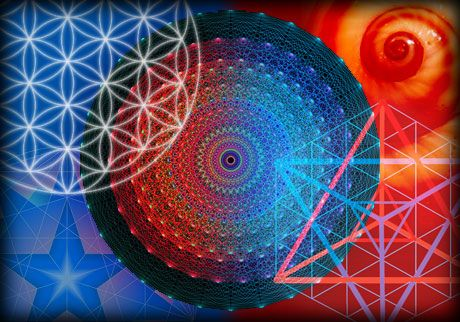Math and Music Studio

# New ClassesMath & Music Studio’s 2018-2019 Course Offerings

Math Seminar: The Homology and Cohomology Theories of Topology

The Homology and cohomology theories of topology are modern methods for counting the number of holes in high dimensional real and complex spaces. These theories count the number of holes in a topological space and allow mathematicians to connect geometry, analysis (calculus), and abstract algebra in useful ways. In terms of applications, the notions of homology and cohomology are rooted in electrodynamics. Cohomology first appeared in quantum physics in the late 1960’s and currently plays a role in condensed matter theory, quantum guage theories and general relativity. Other uses for homology and cohomology include artificial intelligence, big topological data analysis, neuroscience, and vision and sensor networks. The seminar’s topics include simplicial and CW complexes, free Abelian groups, chain complexes and boundary operators, homology groups of surfaces, invariance of homology groups, singular homology, Eilenberg-Steenrod axioms, tensor products and Kunneth Formula, the cohomology ring, and cup and cap products.

Prerequisite: Point Set Topology or Real Analysis

Classical Euclidean Geometry

The primary focus of this course in geometry is on the basic theorems of Euclid. Students will present their own proofs of the major and related theorems in class each week. Topics to be presented include parallel lines, transversals, triangles, congruence, perpendicular bisectors, parallelogram theorems, trapezoids, similarity, right triangles, trigonometry, Pythagorean theorem, inscribed angle theorems, polygons, area and perimeter.

Prerequisite: Algebra 1

Algebra 2 and Trigonometry

This honors level course builds on the foundations laid in algebra 1 and geometry, and stresses student mastery of analysis and graphing of polynomials, logarithmic, exponential and trigonometric functions. Topics include linear equations, matrices, determinants, polynomials, rational functions, imaginary and complex numbers, quadratic equations, inequalities, trigonometry, vectors, polar coordinates, conic sections, circles, parabolas, ellipses and hyperbolas. Students who complete this course will then be prepared to study Differential and Integral Calculus.

Prerequisite: Algebra 1 and Geometry

Differential and Integral Calculus with Differential Equations

This accelerated course is the equivalent of taking both AB and BC calculus, combined with an introduction to differential equations in the spring semester. Calculus is the mathematical study of change and its two major branches, differential and integral, are related to each other by the fundamental theorem of calculus. Today, calculus has broad applications in science, mathematics, engineering, and economics. The calculus topics in this course include limits, derivatives, related rates, mean value theorem, optimization, integrals, fundamental theorem of calculus, volume, surface area, infinite sequences and series, power series, and Taylor series.

The differential equations portion will focus on the unifying concept of dynamical systems. Examples of dynamical systems include things that change over time, such as wind, weather, ocean currents, planetary motion, pendulums and mass spring systems. Topics will include first order linear differential equations, vector spaces, vector fields, harmonic oscillator equations, eigenvalues, and techniques of Euler and Lagrange for solving second order linear differential equations.

Prerequisite

: Algebra 2 and Trigonometry

Multivariable Calculus and Introduction to Real Analysis

Multivariable calculus extends calculus to several variables. Many physical quantities depend on more than one variable. For example, in thermodynamics, pressure depends on both volume and temperature, and modeling fluid flow depends on position and time. The multivariable calculus topics in this course include parametric curves, partial derivatives, gradients and extrema, Fubini’s theorem, double and triple integrals, Green’s theorem, line integrals, Stokes’ theorem, and surface integrals.

Real analysis is the theoretical version of differential and integral calculus from a rigorous point of view, and it helps students develop the ability to think deductively and create their own mathematical proofs. In the real analysis portion of this course, students will study the real number system, real topological spaces, compactness, connectedness, completeness, set theory, Archemedian properties, Bolzano-Weierstrass theorem, Heine-Borel theorem, convergence, continuous functions, and Cauchy sequences.

Prerequisite: Differential and Integral Calculus

Math & Music Studio’s music courses are designed for gifted learners who are interested in delving deeper into aspects of music theory and composition, and improving their appreciation and mastery of music. The instructor, John Rosasco, is a hit songwriter, producer, arranger, jazz pianist and film composer with four Grammy nominations to his credit.

Advanced Music Theory and Composition is being offered as a third year course for our continuing music students, as well as other young musicians who’ve previously studied music theory and want to improve their composition skills. Topics in this course include singing augmented/diminished scales and modes so they become automatic and natural to the listener, melodic dictation, advanced ear training exercises, reharmonization techniques, melodic development, transposition, exploring various music styles, analyzing aspects of iconic works of influential composers, improvisation, polyrhythms, pointillism, open/closed voicings, and polytonality. Additionally, the class will analyze the role and function of music in film and television, and learn how composers use musical elements and expressive devices to create images and evoke emotions. Students will write original pieces each week and receive encouraging and constructive feedback from the instructor. Mutual respect for each person’s work will set the tone in this class.

Prerequisite: A working knowledge of music theory and some experience playing a musical instrument. The ability to play the piano or use music composition software will benefit students taking this course.